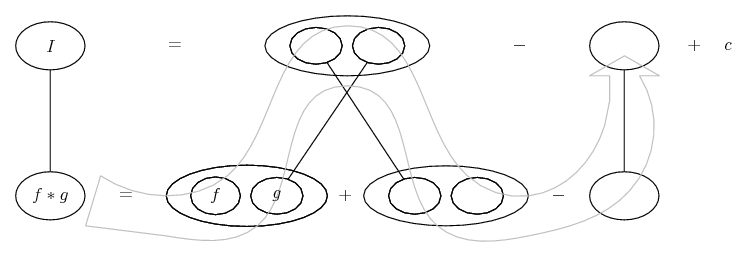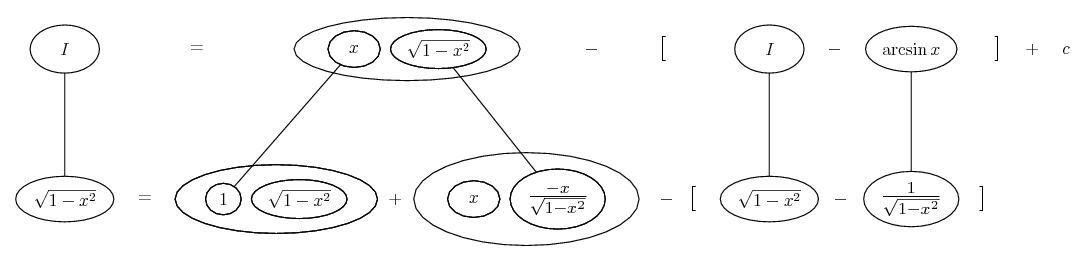# Thread: integration (squareroot)

1. ## integration (squareroot)

What would be the best/quickest way to integrate

squareroot(1-x^2) ?

2.Originally Posted by gomesWhat would be the best/quickest way to integrate

squareroot(1-x^2) ?
1. Use integration by parts:

$\displaystyle \int(\sqrt{1-x^2}) dx = \int(1 \cdot \sqrt{1-x^2}) dx$

2. In the second step keep in mind that

$\displaystyle \int \left(\frac1{\sqrt{1-x^2}}\right) dx = \arcsin(x)$

3.Originally Posted by gomesWhat would be the best/quickest way to integrate

squareroot(1-x^2) ?

Substitute $\displaystyle \sin t=x$ ...Tonio

4.Originally Posted by earboth1. Use integration by parts:

$\displaystyle \int(\sqrt{1-x^2}) dx = \int(1 \cdot \sqrt{1-x^2}) dx$

2. In the second step keep in mind that

$\displaystyle \int \left(\frac1{\sqrt{1-x^2}}\right) dx = \arcsin(x)$

I don't think the above helps: $\displaystyle u=\sqrt{1-x^2}\Longrightarrow u'=-\frac{x}{\sqrt{1-x^2}}\,,\,\,v'=1\Longrightarrow v=x$ , so we get the integral $\displaystyle \int\frac{x^2}{\sqrt{1-x^2}}\,dx$ ...which not only is not an arcsine but it doesn't look easy at all...

Unless, of course, I missed something.

Tonio

5.Originally Posted by gomesWhat would be the best/quickest way to integrate

squareroot(1-x^2) ?
Trigonometric or Hyperbolic substitution works well...

Let $\displaystyle x = \sin{\theta}$ so that $\displaystyle dx = \cos{\theta}\,d\theta$.

The integral becomes

$\displaystyle \int{\sqrt{1 - x^2}\,dx} = \int{\sqrt{1 - \sin^2{\theta}}\,\cos{\theta}\,d\theta}$

$\displaystyle = \int{\cos{\theta}\cos{\theta}\,d\theta}$

$\displaystyle = \int{\cos^2{\theta}\,d\theta}$

$\displaystyle = \int{\frac{1}{2}\cos{2\theta} + \frac{1}{2}\,d\theta}$

$\displaystyle = \frac{1}{4}\sin{2\theta} + \frac{1}{2}\theta + C$

$\displaystyle = \frac{2\sin{\theta}\cos{\theta}}{4} + \frac{\theta}{2} + C$

$\displaystyle = \frac{\sin{\theta}\cos{\theta} + \theta}{2} + C$.

Remembering that $\displaystyle x = \sin{\theta}$, that means $\displaystyle \cos{\theta} = \sqrt{1 - x^2}$ and $\displaystyle x = \arcsin{\theta}$ and substituting, we find

$\displaystyle \int{\sqrt{1 - x^2}\,dx} = \frac{x\sqrt{1 - x^2} + \arcsin{x}}{2} + C$.

6.Originally Posted by tonioUnless, of course, I missed something.

Tonio
I think earboth is going to re-write

$\displaystyle \frac{-x^2}{\sqrt{1 - x^2}}$

as

$\displaystyle \frac{1 - x^2 - 1}{\sqrt{1 - x^2}}\ =\ \sqrt{1 - x^2}\ -\ \frac{1}{\sqrt{1 - x^2}}$

and then solve the top row of...... for I. Which is no longer, really.

Key:
Spoiler:... is lazy integration by parts, doing without u and v.... is the product rule, straight continuous lines differentiating downwards (integrating up) with respect to x.

Full size:__________________________________________________ ___
Don't integrate - balloontegrate!

Balloon Calculus; standard integrals, derivatives and methods

Balloon Calculus Drawing with LaTeX and Asymptote!

7. Thanks everyone, really appreciate it.#### Search Tags

integration, squareroot